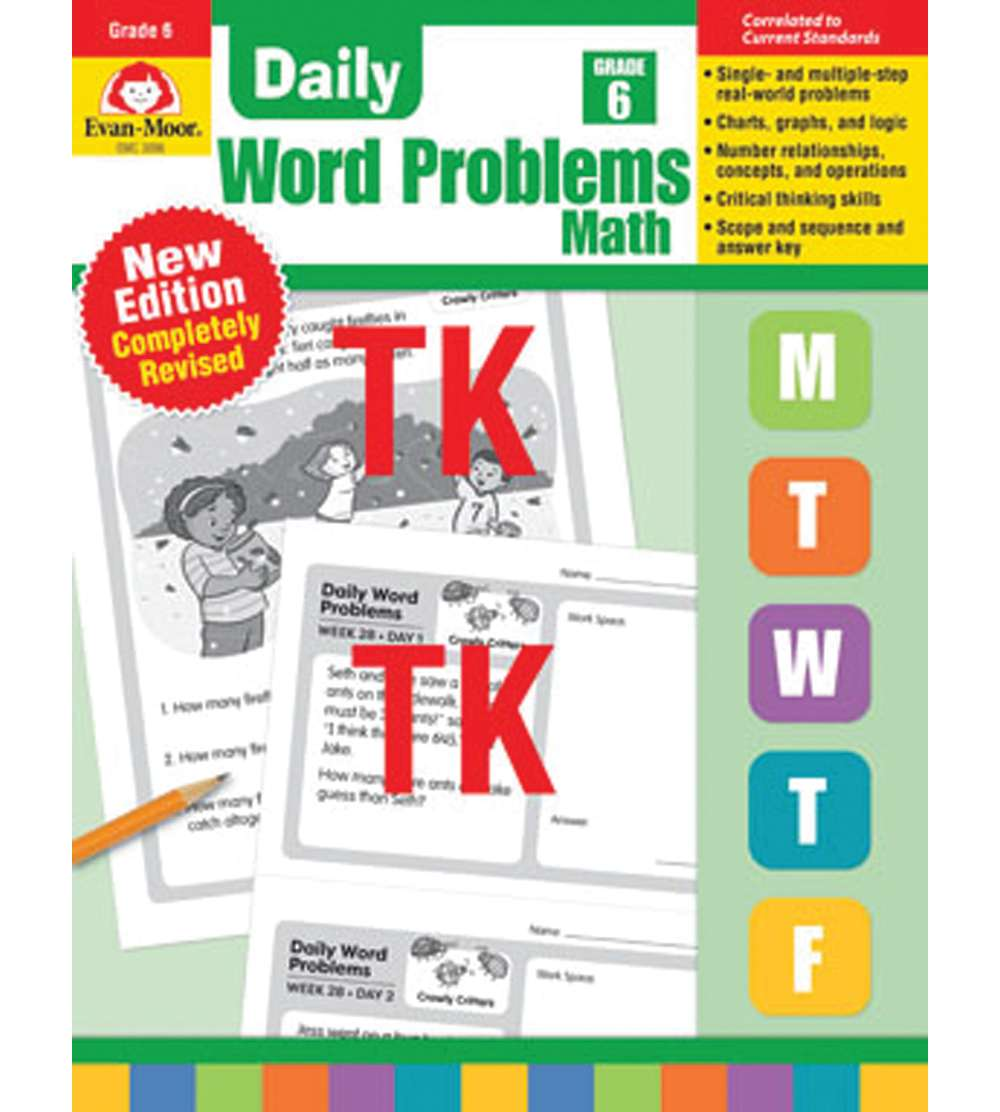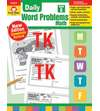•# Daily Word Problems Math, Grade 6

Product Number: EMC3096
You must be logged
\$22.99
Completely revised in 2019 to reflect grade-level standards, Daily Word Problems is the perfect resource to improve students’ problem-solving skills. The all-NEW word problems are written to support current math standards and expectations and provide consistent spiral review of math concepts. Students’ problem-solving skills improve as they participate in meaningful, real-life math practice.

The 36 weeks of activities provide practice of grade-level math concepts such as addition, multiplication, fractions, logic, algebra, and more.

Each week focuses on a theme and provides a daily word problem within the context of a real-life situation.
• Monday–Thursday’s activities provide a one- or two-step word problem.
• Friday’s format is more extensive and requires multiple steps. The multi-step problems require students to incorporate higher-order thinking skills by applying their understanding within a different context.
• Reproducible pages provide plenty of room for students to solve using the strategy of their choice.
What’s new in the revised edition of Daily Word Problems?
• All-new word problems to support current standards.
• New weekly themes that present problems in the context of real life
• Day-by-day skills list to help teachers identify skills practiced
Grade 6 math skill practice includes:
• positive and negative numbers
• multiplication and division
• factors and multiples
• fractions
• decimals
• percentages
• ratios and rates
• inequalities
• time and temperature
• linear measurement
• weight and capacity
• perimeter and area
• surface area and volume
• statistics
• coordinate plane
• graphs, charts, and maps
• logical thinking
• spatial reasoning
• expressions and equations
Includes scope and sequence chart and an answer key.
Quantity

Completely revised in 2019 to reflect grade-level standards, Daily Word Problems is the perfect resource to improve students’ problem-solving skills. The all-NEW word problems are written to support current math standards and expectations and provide consistent spiral review of math concepts. Students’ problem-solving skills improve as they participate in meaningful, real-life math practice.

The 36 weeks of activities provide practice of grade-level math concepts such as addition, multiplication, fractions, logic, algebra, and more.

Each week focuses on a theme and provides a daily word problem within the context of a real-life situation.
• Monday–Thursday’s activities provide a one- or two-step word problem.
• Friday’s format is more extensive and requires multiple steps. The multi-step problems require students to incorporate higher-order thinking skills by applying their understanding within a different context.
• Reproducible pages provide plenty of room for students to solve using the strategy of their choice.
What’s new in the revised edition of Daily Word Problems?
• All-new word problems to support current standards.
• New weekly themes that present problems in the context of real life
• Day-by-day skills list to help teachers identify skills practiced
Grade 6 math skill practice includes:
• positive and negative numbers
• multiplication and division
• factors and multiples
• fractions
• decimals
• percentages
• ratios and rates
• inequalities
• time and temperature
• linear measurement
• weight and capacity
• perimeter and area
• surface area and volume
• statistics
• coordinate plane
• graphs, charts, and maps
• logical thinking
• spatial reasoning
• expressions and equations
Includes scope and sequence chart and an answer key.
EMC3096

### Data sheet

Manufacturer
Evan-Moor Corporation